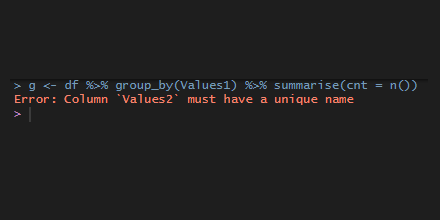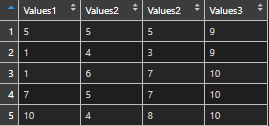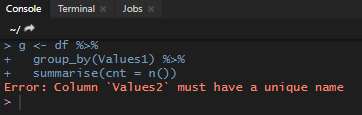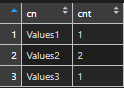# dplyr error: column must have a unique name

In rare cases, the data source may contain multiple columns with the same name, and some of the next actions with dplyr functions might result in the error “column must have unique name”.

Here is a data frame with columns that have the same name.

```df <- data.frame(
Values1 = sample(1:10, 5, replace=T),
Values2 = sample(1:10, 5, replace=T),
Values2 = sample(1:10, 5, replace=T),
Values3 = sample(1:10, 5, replace=T),
check.names = FALSE
)```If I want to group and summarize something, I get this error.

```g <- df %>%
group_by(Values1) %>%
summarise(cnt = n())```## Prevent dplyr error: column must have a unique name

To prevent this dplyr error, you have to rename some of the data frame columns.

In large data frames, a summary of data frame column names might be handy. By using that you can detect which of the column names is more than once.

```name_count <- data.frame(cn = names(df)) %>%
group_by(cn) %>%
summarize(cnt = n())```## My top 10 favorite dplyr tips and tricks

If you like to work with the dplyr package, then here are helpful tips and tricks.

1. Rename columns by using the dplyr select function
2. Calculate in row context with dplyr
3. Rearrange columns quickly with dplyr everything
4. Drop unnecessary columns with dplyr
5. Use dplyr count or add_count instead of group_by and summarize
6. Replace nested ifelse with dplyr case_when function
7. Execute calculations across columns conditionally with dplyr
8. Filter by calculation of grouped data inside filter function
9. Get top and bottom values by each group with dplyr
10. Reflow your dplyr code

Categories R